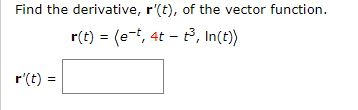# Find the derivative, r'(t), of the vector function. r(t) = (e-t, 4t - t, In(t) r'(t)

Questionhelp_outlineImage TranscriptioncloseFind the derivative, r'(t), of the vector function. r(t) = (e-t, 4t - t, In(t) r'(t) fullscreen

1 Rating

### Want to see this answer and more?

Experts are waiting 24/7 to provide step-by-step solutions in as fast as 30 minutes!*

*Response times vary by subject and question complexity. Median response time is 34 minutes and may be longer for new subjects.
Tagged in
MathCalculus

### Applications of Derivative